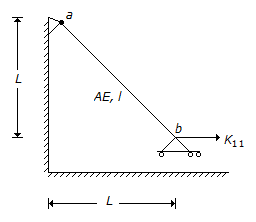# Civil Engineering - GATE Exam Questions - Discussion

Discussion Forum : GATE Exam Questions - Section 6 (Q.No. 4)
4.
Horizontal stiffness coefficient, K11 of bar ab is given by :A E/l 2
A E/2 1
A E/l
2 A E/l
Explanation:
No answer description is available. Let's discuss.
Discussion:
3 comments Page 1 of 1.

MUKESH RANWA said:   2 years ago
Horizontal force = p*2^1/2.
Horizontal displacement = δ/2^1/2.
K11 = h.f/h.d.
δ = pl/AE,
K11 = 2AE/l.

Dhanapm said:   5 years ago
k=force in the member to unit deflection.
k=(p/sqrt2)/(pl/AE).

Gaja said:   6 years ago